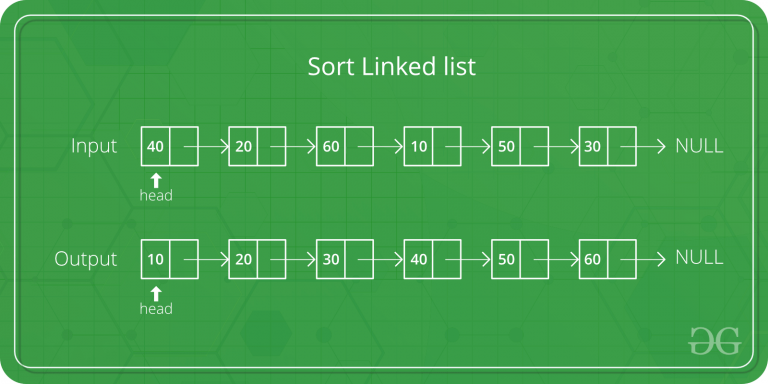# C++ Program For QuickSort On Singly Linked List

• Difficulty Level : Medium
• Last Updated : 23 Dec, 2021

QuickSort on Doubly Linked List is discussed here. QuickSort on Singly linked list was given as an exercise. The important things about implementation are, it changes pointers rather swapping data and time complexity is same as the implementation for Doubly Linked List.In partition(), we consider last element as pivot. We traverse through the current list and if a node has value greater than pivot, we move it after tail. If the node has smaller value, we keep it at its current position.

In QuickSortRecur(), we first call partition() which places pivot at correct position and returns pivot. After pivot is placed at correct position, we find tail node of left side (list before pivot) and recur for left list. Finally, we recur for right list.

## C++

 `// C++ program for Quick Sort on ``// Singly Linked List``#include ``#include ``using` `namespace` `std;`` ` `// A node of the singly ``// linked list ``struct` `Node ``{``    ``int` `data;``    ``struct` `Node* next;``};`` ` `/* A utility function to insert a ``   ``node at the beginning of ``   ``linked list */``void` `push(``struct` `Node** head_ref, ``          ``int` `new_data)``{``    ``// Allocate node ``    ``struct` `Node* new_node = ``new` `Node;`` ` `    ``// Put in the data ``    ``new_node->data = new_data;`` ` `    ``// Link the old list off the ``    ``// new node ``    ``new_node->next = (*head_ref);`` ` `    ``// Move the head to point to ``    ``// the new node ``    ``(*head_ref) = new_node;``}`` ` `// A utility function to print ``// linked list ``void` `printList(``struct` `Node* node)``{``    ``while` `(node != NULL) ``    ``{``        ``printf``(``"%d "``, node->data);``        ``node = node->next;``    ``}``    ``printf``(``""``);``}`` ` `// Returns the last node of the list``struct` `Node* getTail(``struct` `Node* cur)``{``    ``while` `(cur != NULL &&``           ``cur->next != NULL)``        ``cur = cur->next;``    ``return` `cur;``}`` ` `// Partitions the list taking the ``// last element as the pivot``struct` `Node* partition(``struct` `Node* head, ``                       ``struct` `Node* end,``                       ``struct` `Node** newHead,``                       ``struct` `Node** newEnd)``{``    ``struct` `Node* pivot = end;``    ``struct` `Node *prev = NULL, ``                ``*cur = head, *tail = pivot;`` ` `    ``// During partition, both the head and ``    ``// end of the list might change which ``    ``// is updated in the newHead and newEnd ``    ``// variables``    ``while` `(cur != pivot) ``    ``{``        ``if` `(cur->data < pivot->data) ``        ``{``            ``// First node that has a value ``            ``// less than the pivot - becomes ``            ``// the new head``            ``if` `((*newHead) == NULL)``                ``(*newHead) = cur;`` ` `            ``prev = cur;``            ``cur = cur->next;``        ``}`` ` `        ``// If cur node is greater than pivot``        ``else` `        ``{``            ``// Move cur node to next of tail, ``            ``// and change tail``            ``if` `(prev)``                ``prev->next = cur->next;``            ``struct` `Node* tmp = cur->next;``            ``cur->next = NULL;``            ``tail->next = cur;``            ``tail = cur;``            ``cur = tmp;``        ``}``    ``}`` ` `    ``// If the pivot data is the smallest element ``    ``// in the current list, pivot becomes the head``    ``if` `((*newHead) == NULL)``        ``(*newHead) = pivot;`` ` `    ``// Update newEnd to the current last node``    ``(*newEnd) = tail;`` ` `    ``// Return the pivot node``    ``return` `pivot;``}`` ` `// here the sorting happens exclusive of the ``// end node``struct` `Node* quickSortRecur(``struct` `Node* head,``                            ``struct` `Node* end)``{``    ``// Base condition``    ``if` `(!head || head == end)``        ``return` `head;`` ` `    ``Node *newHead = NULL, *newEnd = NULL;`` ` `    ``// Partition the list, newHead and newEnd ``    ``// will be updated by the partition function``    ``struct` `Node* pivot = partition(head, end, ``                                   ``&newHead, &newEnd);`` ` `    ``// If pivot is the smallest element - no need ``    ``// to recur for the left part.``    ``if` `(newHead != pivot)``    ``{``        ``// Set the node before the pivot node ``        ``// as NULL``        ``struct` `Node* tmp = newHead;``        ``while` `(tmp->next != pivot)``            ``tmp = tmp->next;``        ``tmp->next = NULL;`` ` `        ``// Recur for the list before pivot``        ``newHead = quickSortRecur(newHead, tmp);`` ` `        ``// Change next of last node of the ``        ``// left half to pivot``        ``tmp = getTail(newHead);``        ``tmp->next = pivot;``    ``}`` ` `    ``// Recur for the list after the ``    ``// pivot element``    ``pivot->next = quickSortRecur(pivot->next, ``                                 ``newEnd);`` ` `    ``return` `newHead;``}`` ` `// The main function for quick sort. ``// This is a wrapper over recursive ``// function quickSortRecur()``void` `quickSort(``struct` `Node** headRef)``{``    ``(*headRef) = quickSortRecur(*headRef, ``                                 ``getTail(*headRef));``    ``return``;``}`` ` `// Driver code``int` `main()``{``    ``struct` `Node* a = NULL;``    ``push(&a, 5);``    ``push(&a, 20);``    ``push(&a, 4);``    ``push(&a, 3);``    ``push(&a, 30);`` ` `    ``cout << ``"Linked List before sorting "``;``    ``printList(a);`` ` `    ``quickSort(&a);`` ` `    ``cout << ``"Linked List after sorting "``;``    ``printList(a);`` ` `    ``return` `0;``}`

Output:

```Linked List before sorting
30 3 4 20 5